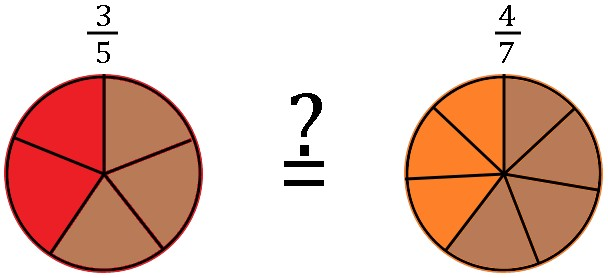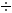Compare Fractions

Compare Fractions

Suppose that you made a cake, and you want to give  of the cake to your friend, but then you learn that your friend took  of the cake. So, did your friend take more, less, or the exact amount that you wanted to give him?  We can draw both fractions:By looking at the figure, it seems that both fractions are the same, but is this the case? We need a better way to compare these fractions.

There are two ways to compare these fractions:

I.  Find a Common Denominator

To find the answer, we can compare the numerators for both fractions by converting their denominators to the same value.

Example

Are and the same or is one greater

Solution

First multiply the values on one side by the denominator on the opposite side

?

Now that both denominators are the same, compare numerators

?

?

From here we can see that 3/5(or 21/35) > 4/7(or 20/35) since 21 > 20

>

II.  Convert Fractions to Decimals

In the example above divide the numerator by the denominator

?

35 ? 47

Compare both values

0.6 >

So, now you know that your friend did not take as much cake as you wanted to give him.

Try it yourself

Order the following fractions from least to greatest.

1.)

2.)

3.) 1 3

Insert <, > or = to make to the following statements true.

4.)  ___

5.)  2 ___ 3

6.)  ___ 3

7.)  ___